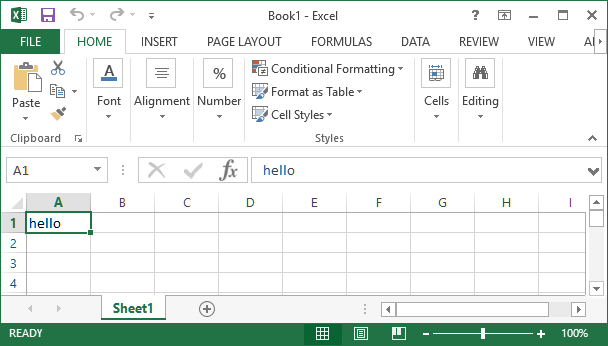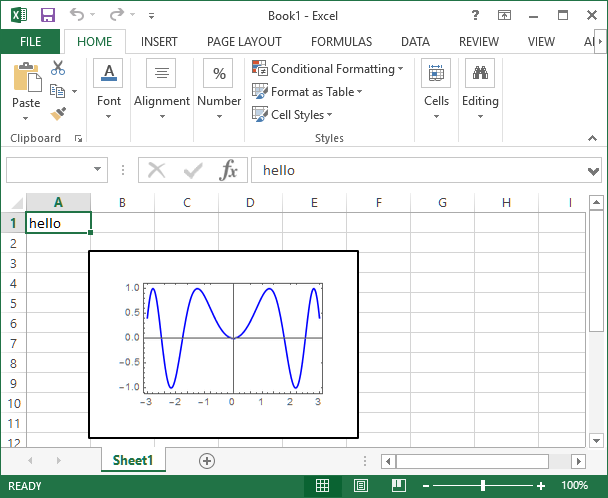# How do I get started using Excel from Mathematica?

Mathematica Link for Excel enables you to share data and calculations between Mathematica and Microsoft Excel.

Here we showcase the first few steps in using ExcelLink to connect to Excel from Mathematica. For connecting to Mathematica from Excel, see here.

Advanced information can be found in the Mathematica Link for Excel documentation.

``<< ExcelLink`` `

This loads a library of functions and symbols into Mathematica.

### Writing Text or Data to an Excel Sheet

Assign the value to the `Excel` function with the Excel cell ID as the argument. This puts the string `"hello"` in cell A1 in the active spreadsheet.

``Excel["A1"] = "hello" ``### Placing a Graphic in Excel

This creates a graphic in Mathematica and assigns it to a cell. The upper-left corner of this plot appears in cell B3.

``````g = Plot[Sin[a^2], {a, -3, 3}, PlotStyle -> Blue, Frame -> True, ImageSize -> 200];
Excel["B3"] = g ``````### Reading from an Excel sheet

Data from an Excel spreadsheet can be read into Mathematica using the `Excel` function and the range of addresses.

``Excel["A2:A6"]``

Note that numerical data is read as floating-point (real) numbers.

### Saving an Excel Sheet

Changes in an Excel spreadsheet can be saved from Mathematica using the `ExcelSave[]` function.

## Contact SupportCall us

## Customer Support

Monday–Friday
8am–5pm US Central Time

• Product registration or activation
• Pre-sales information and ordering
• Help with installation and first launch

## Advanced Technical Support (for eligible customers)

Monday–Thursday
8am–7pm US Central Time

Friday
8:30–10am & 11am–5pm US Central Time

• Priority technical support
• Product assistance from Wolfram experts
• Help with Wolfram Language programming